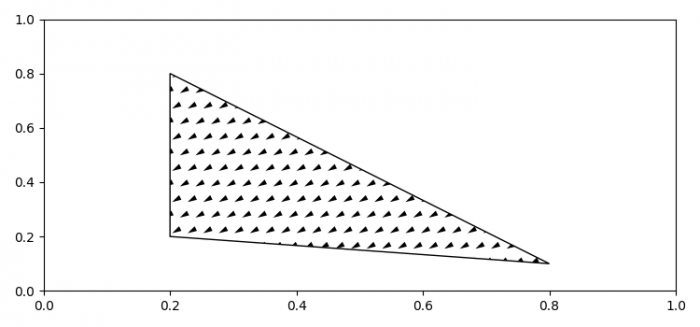# How to fill a polygon with a custom hatch in Matplotlib?

To fill a polygon with a custom hatch in matplotlib, we can override the matplotlib.hatch.Shapes class.

## Steps

• Set the figure size and adjust the padding between and around the subplots.
• Make a hatch shape using polygon class and get the path.
• Override the custom hatch shape class, with shape_vertices, shape_codes, etc.
• Create a new figure or activate an existing figure.
• Add an axes to the figure as part of a subplot arrangement.
• Add an axes patch of shape polygon.
• Set the hatching pattern.
• To display the figure, use show() method.

## Example

import matplotlib.hatch
import matplotlib.pyplot as plt
from matplotlib.patches import Polygon

plt.rcParams["figure.figsize"] = [7.50, 3.50]
plt.rcParams["figure.autolayout"] = True

my_hatch_shape = Polygon(
[[0., 0.4], [-0.3, 0.1], [0.1, 0.2]],
closed=True, fill=False).get_path()

class MyCustomHatch(matplotlib.hatch.Shapes):
filled = True
size = 1.0
path = my_hatch_shape

def __init__(self, hatch, density):
self.num_rows = (hatch.count('c')) * density
self.shape_vertices = self.path.vertices
self.shape_codes = self.path.codes
matplotlib.hatch.Shapes.__init__(self, hatch, density)

matplotlib.hatch._hatch_types.append(MyCustomHatch)

fig = plt.figure()

plt.show()